Cross Reference Down

Diaconis, P (2002). G.H. Hardy and Probability ???. Bulletin of the London Mathematical Society 34(4), pp. 385-402.

This work cites the following items of the Benford Online Bibliography:

 Diaconis, P (1977). The Distribution of Leading Digits and Uniform Distribution Mod 1. Annals of Probability 5(1), pp. 72-81. ISSN/ISBN:0091-1798.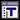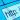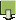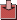Feller, W (1971). An Introduction to Probability Theory and Its Applications. 2nd ed., J. Wiley (see p 63, vol 2).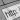Flehinger, BJ (1966). On the Probability that a Random Integer has Initial Digit A. American Mathematical Monthly 73(10), pp. 1056-1061. ISSN/ISBN:0002-9890. DOI:10.2307/2314636.Hill, TP (1995). A Statistical Derivation of the Significant-Digit Law. Statistical Science 10(4), pp. 354-363. ISSN/ISBN:0883-4237.Knuth, DE (1997). The Art of Computer Programming. pp. 253-264, vol. 2, 3rd ed, Addison-Wesley, Reading, MA.Raimi, RA (1976). The First Digit Problem. American Mathematical Monthly 83(7), pp. 521-538. ISSN/ISBN:0002-9890. DOI:10.2307/2319349.